### Home > PC > Chapter 6 > Lesson 6.2.1 > Problem6-75

6-75.
1. Solve the following equations. Homework Help ✎

1. x2 − 4x = 21

2. (x − 2)(x + 1) = 4

3. 3 +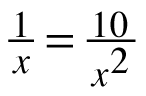4.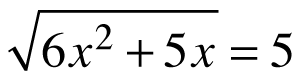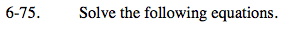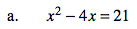1. Set everything = 0
2. Factor or use the quadratic formula.
3. How many answers should you have?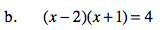Set the entire function = 0.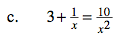Multiply each term by x2.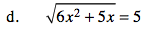Square both sides first.